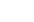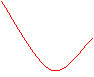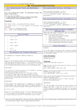How to Learn in 24 Hours?The Rapid Learning Movie

 Need Help? M-F: 9am-5pm(PST): Toll-Free: (877) RAPID-10 US Direct: (714) 692-2900 Int'l: 001-714-692-2900 24/7 Online Technical Support: The Rapid Support Center Secure Online Order:Need Proof? Testimonials by Our Users

 Rapid Learning Courses: MCAT in 24 Hours (2015-16) USMLE in 24 Hours (Boards) Chemistry in 24 Hours Biology in 24 Hours Physics in 24 Hours Mathematics in 24 Hours Psychology in 24 Hours SAT in 24 Hours ACT in 24 Hours AP in 24 Hours CLEP in 24 Hours DAT in 24 Hours (Dental) OAT in 24 Hours (Optometry) PCAT in 24 Hours (Pharmacy) Nursing Entrance Exams Certification in 24 Hours eBook - Survival Kits Audiobooks (MP3)

 Tell-A-Friend: Have friends taking science and math courses too? Tell them about our rapid learning system.Home »  Mathematics »  Introductory Statistics

Scatterplots and Correlation

 Topic Review on "Title": Response Variable: is the y-variable that measures the outcome of the study. Explanatory Variable: is the x-variable that “explains” or predicts the observed outcome. Scatterplot: is a graph that shows the relationship between two quantitative variables measured on the same individual. Correlation: numerical measure of the strength and direction of the linear relationship between two quantitative variables, written as r. r =(Σzxzy)/(n-1), correlation coefficient Scatterplots use distinct variables on each axis The x-axis is used to plot the explanatory variable. The y-axis is used to plot the response variable. The x-variable explains the y-variable If there is no distinction between the two variables, either can go on the x-axis. In a scatterplot, each individual is a point corresponding to the x and y values of both variables. Aid in understanding how one variable affects another. Enable us to detect patterns or trends. Help to visually determine the strength of of the relationship. Have direction, form and strength. Scatterplots: Direction Positively Associated acatterplots show an increase in y, whenever there is an increase in x. Pattern extends from the bottom left of the graph to the upper right Negatively Associated Scatterplots, show a decrease in y, whenever there is an increase in x. Pattern extends from the upper left to bottom right.  Sometimes there is no clear direction, therefore you can make no distinction about the part of a scatterplot pattern.PositiveNegativeNo   Scatterplots Form: A scatterplot is linear if it has points that lie in a straight line pattern.A scatterplot is curved or non-linear if the relationship is not straight.LineCurved

Rapid Study Kit for "Title":
 Flash Movie Flash Game Flash Card Core Concept Tutorial Problem Solving Drill Review Cheat Sheet"Title" Tutorial Summary : This tutorial describes what scatterplots and correlation are and their properties.  First, we identify the role of variables on the x and y axis then we identify and create scatterplots and describe the overall pattern of a scatterplot using direction, form and strength. We also identify and describe outliers and use correlation to describe the relationship between two variables. Finally, we will compute correlation of two quantitative variables so you will know the conditions of correlation and how to apply them.

 Tutorial Features: Specific Tutorial Features: Step by step examples are shown to introduce scatterplots and correlation. Details and illustrative graphs on how to identify pattern, form and strength of scatterplots are provided. The relationship between z-score and correlation coefficient and details as to how to calculate the latter are also provided. Series Features: Concept map showing inter-connections of new concepts in this tutorial and those previously introduced. Definition slides introduce terms as they are needed. Visual representation of concepts Animated examples—worked out step by step A concise summary is given at the conclusion of the tutorial.

 "Title" Topic List: Response Variable Explanatory Variable Scatterplots Correlation

See all 24 lessons in Introductory Statistics, including concept tutorials, problem drills and cheat sheets:
Teach Yourself Introductory Statistics Visually in 24 Hours